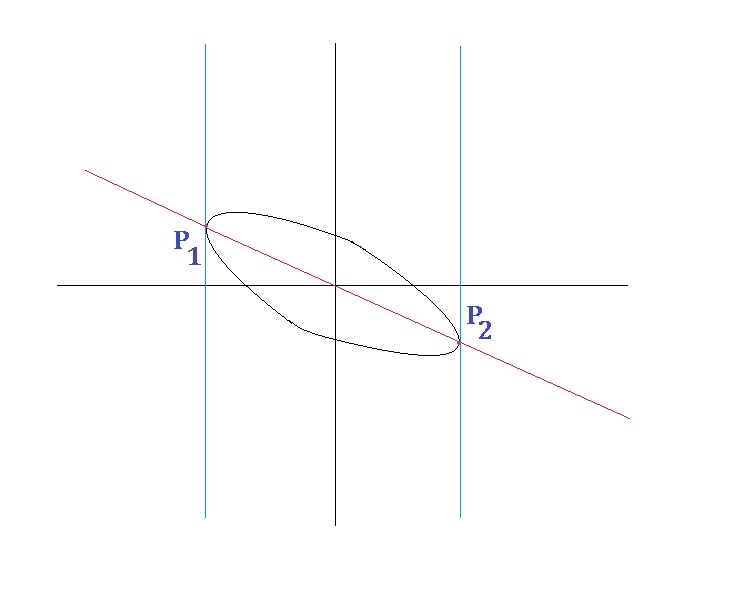# Rolling Rugby

Calculus Level 2As shown above is the elliptical graph of $x^2 + xy + y^2 = 7$, where $P_{1}$ and $P_{2}$ are the points on the graph with minimum and maximum $x$ coordinates respectively, and the red line $l$ is the linear graph passing through $P_{1}$ and $P_{2}$.

If the tangent parallel to line $l$ touches the graph at a point $P_{3} (a,b)$ for some real numbers $a,b$, compute $a^2 + b^2$.

×

Problem Loading...

Note Loading...

Set Loading...